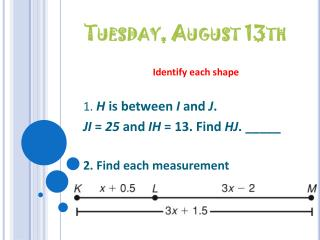DownloadDownload PresentationTuesday, August 13th

# Tuesday, August 13th

Download Presentation## Tuesday, August 13th

- - - - - - - - - - - - - - - - - - - - - - - - - - - E N D - - - - - - - - - - - - - - - - - - - - - - - - - - -
##### Presentation Transcript

1. Tuesday, August 13th Identify each shape 1. H is between I and J. JI = 25 and IH = 13. Find HJ. _____ 2. Find each measurement

2. Homework Answers Please ask if you have questions!

3. Angle Exploration Need: • Paper • Protractor • Lined sheet of paper

4. Discussion 7. What did you notice about the measure of <ABC? 8. What was the relationship between measures of <ABC, <ABD, and <DBC? Always 90˚ m<ABD + m<DBC=m<ABC

5. Part 1: Naming Angles

6. An angleis a figure formed by two rays, or sides, with a common endpoint called the vertex(plural: vertices). You can name an angle several ways: by its vertex by a point on each ray and the vertex by a number.

7. The set of all points between the sides of the angle is the interior of an angle. The exterior of an angleis the set of all points outside the angle. Angle Name R, SRT, TRS, or 1

8. Example 1 A surveyor recorded the angles formed by a transit (point A) and three distant points, B, C, and D. Name three of the angles. Possible answer: BAC CAD BAD

9. Example 2 Write the different ways you can name the angles in the diagram. RTQ, T, STR, 1, 2

10. Part 2: Measuring Angles

11. The measureof an angle is usually given in degrees. Since there are 360° in a circle, one degreeis of a circle. When you use a protractor to measure angles, you are applying the following postulate.

12. Practice! Draw 2 angles and measure with your protractor!

13. Reminder! Congruent angles are angles that have the same measure. In the diagram, mABC = mDEF, so you can write ABC  DEF. This is read as “angle ABC is congruent to angle DEF.” Arc marks are used to show that the two angles are congruent.

14. Part 3: Constructing Angles http://www.youtube.com/watch?v=YphAYfe6J40

15. Example #1- Let’s try together! Use your notes from Friday!!!! “sharp point” = Bullseye end of compass

16. Example #2- You Try! Use your notes from Friday!!!! “sharp point” = Bullseye end of compass

17. Homework Practice A Worksheet